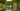# JavaScript 中克隆数组的方法

JavaScript 可以做很多事情，现在我们了解处理数组克隆。

## 扩展操作符（浅拷贝）

``````const numbers = [1, 2, 3];
const numbersCopy = [...numbers];``````

``````numbersCopy.push(4);
console.log(numbers, numbersCopy);
// [1, 2, 3] and [1, 2, 3, 4]
// numbers 是独立的``````

``````const nestedNumbers = [, ];
const numbersCopy = [...nestedNumbers];

numbersCopy.push(300);
console.log(nestedNumbers, numbersCopy);
// [[1, 300], ]
// [[1, 300], ]
// 它们两个都发生改变，因为它们共享引用``````

## for() 循环（浅拷贝）

``````const numbers = [1, 2, 3];
const numbersCopy = [];

for (let i = 0; i < numbers.length; i++) {
numbersCopy[i] = numbers[i];
}``````

``````numbersCopy.push(4);
console.log(numbers, numbersCopy);
// [1, 2, 3] and [1, 2, 3, 4]
// numbers 是独立的``````

``````const nestedNumbers = [, ];
const numbersCopy = [];

for (let i = 0; i < nestedNumbers.length; i++) {
numbersCopy[i] = nestedNumbers[i];
}

numbersCopy.push(300);
console.log(nestedNumbers, numbersCopy);
// [[1, 300], ]
// [[1, 300], ]
// 它们两个都发生改变，因为它们共享引用``````

## while() 循环

`for` 相似。

``````const numbers = [1, 2, 3];
const numbersCopy = [];
let i = -1;

while (++i < numbers.length) {
numbersCopy[i] = numbers[i];
}``````

``````numbersCopy.push(4);
console.log(numbers, numbersCopy);
// [1, 2, 3] and [1, 2, 3, 4]
// numbers 是独立的``````

``````const nestedNumbers = [, ];
const numbersCopy = [];

let i = -1;

while (++i < nestedNumbers.length) {
numbersCopy[i] = nestedNumbers[i];
}

numbersCopy.push(300);
console.log(nestedNumbers, numbersCopy);
// [[1, 300], ]
// [[1, 300], ]
// 它们两个都发生改变，因为它们共享引用``````

## Array.map（浅拷贝）

``````const numbers = [1, 2, 3];
const double = (x) => x * 2;

numbers.map(double);``````

``````const numbers = [1, 2, 3];
numberCopy = numbers.map((x) => x);``````

## Array.filter（浅拷贝）

`Array.filter` 函数像 `map` 那样返回一个数组，但它通常不会生成相同长度数组。

``````[1, 2, 3].filter((x) => x % 2 === 0);
// ``````

``````const numbers = [1, 2, 3];
const numbersCopy = numbers.filter(() => true);``````

## Array.reduce（浅拷贝）

``````const numbers = [1, 2, 3];

const numbersCopy = numbers.reduce((newArray, element) => {
newArray.push(element);

return newArray;
}, []);``````

`Array.reduce` 通过轮询列表来转换初始值。上面的初始值是一个空数组，然后我们使用每一个元素填充它。该数组在回调函数中必须返回以用于下一次的迭代。

## Array.slice（浅拷贝）

`Array.slice` 基于你提供的开始/结束索引返回数组的浅拷贝。如果我们想要开始的 3 个元素：

``````[1, 2, 3, 4, 5].slice(0, 3);
// [1, 2, 3]
// Starts at index 0, stops at index 3
// 在索引 0 开始索引 3 结束``````

``````const numbers = [1, 2, 3, 4, 5];
const numbersCopy = numbers.slice();
// [1, 2, 3, 4, 5]``````

## JSON.parse 和 JSON.stringify（深拷贝）

`JSON.stringify` 转换对象为字符串。`JSON.parse` 转换 JSON 字符串为对象。组合它们可以转换对象为字符串，然后再反向过程会创建一个新数据结构。

``````const nestedNumbers = [, ];
const numbersCopy = JSON.parse(JSON.stringify(nestedNumbers));

numbersCopy.push(300);
console.log(nestedNumbers, numbersCopy);

// [, ]
// [[1, 300], ]
// 这两个数组是完全不同的``````

## Array.concat（浅拷贝）

`Array.cancat` 使用值或其它数组组合数组。

``````[1, 2, 3].concat(4); // [1, 2, 3, 4]
[1, 2, 3].concat([4, 5]); // [1, 2, 3, 4, 5]``````

``````[1, 2, 3].concat(); // [1, 2, 3]
[1, 2, 3].concat([]); // [1, 2, 3]``````

## Array.from（浅拷贝）

`Array.from` 可以转换可迭代对象和类数组对象为数组。

``````const numbers = [1, 2, 3];
const numbersCopy = Array.from(numbers);
// [1, 2, 3]``````